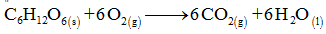# The following unbalanced equation illustrates the overall reaction by which the body utilizes glucose to produce energy:C6H12O6(s) + O2(g) → CO2(g) + H2O(l) What is the conversion factor that allows for the calculation of moles of carbon dioxide based on moles of glucose?

Question
279 views

The following unbalanced equation illustrates the overall reaction by which the body utilizes glucose to produce energy:

C6H12O6(s) + O2(g) → CO2(g) + H2O(l)

What is the conversion factor that allows for the calculation of moles of carbon dioxide based on moles of glucose?

check_circle

star
star
star
star
star
1 Rating
Step 1

The balanced equation for the utilization of glucose to produce energy can be given as...

### Want to see the full answer?

See Solution

#### Want to see this answer and more?

Solutions are written by subject experts who are available 24/7. Questions are typically answered within 1 hour.*

See Solution
*Response times may vary by subject and question.
Tagged in